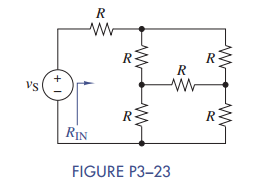### Create an Account

Home / Questions / Use simple engineering intuition to find the input resistance of the circuit in Figure P3...

# Use simple engineering intuition to find the input resistance of the circuit in Figure P3 23 Use either node-voltage or mesh-current analysis to prove your intuition

Use simple engineering intuition to find the input resistance of the circuit in Figure P3−23. Use either node-voltage or mesh-current analysis to prove your intuition.Jun 15 2020 View more View LessSubscribe To Get Solution